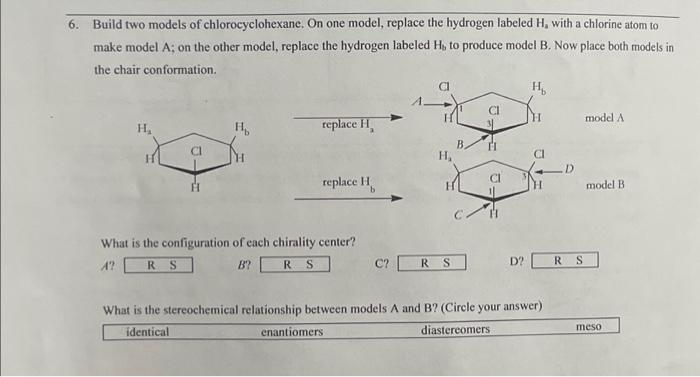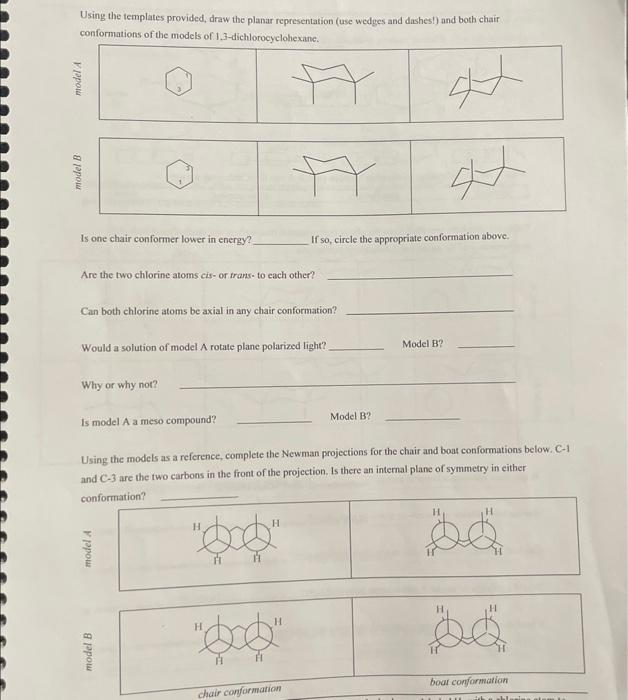# (Solved): please help and explain  Build two models of chlorocyclohexane. On one model, replace the hydro ...Build two models of chlorocyclohexane. On one model, replace the hydrogen labeled $$\mathrm{H}_{2}$$ with a chlorine atom to make model A; on the other model, replace the hydrogen labeled $$\mathrm{H}_{4}$$ to produce model B. Now place both models in the chair conformation. What is the configuration of each chirality center? $$A^{7}$$ ? $$B ?$$ C? D? What is the stereochemical relationship between models A and B? (Circle your answer) Using the templates provided, draw the planar representation (use wedges and dashes!) and both chair conformations of the models of 1,3-dichlorocyclohexane. Is one chair conformer lower in energy? If so, circle the appropriate conformation above. Are the two chlorine atoms cis- or trans- to each other? Can both chlorine atoms be axial in any chair conformation? Would a solution of model A rotate plane polarized light? Model B? Why or why not? Is model A a meso compound? Model B? Using the models as a reference, complete the Newman projections for the chair and boat conformations below. C-1 and $$\mathrm{C}-3$$ are the two carbons in the front of the projection. Is there an internal plane of symmetry in cither